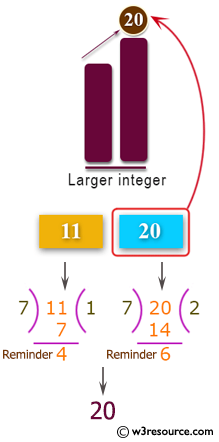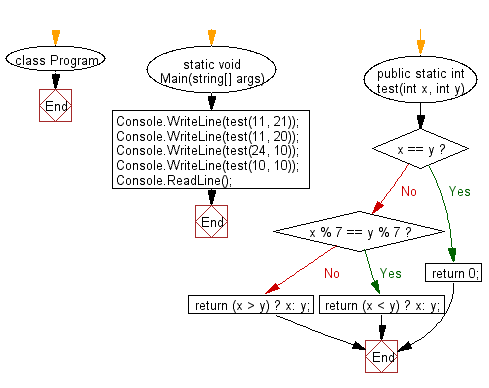﻿ C# - Find the larger from two given integers - w3resource# C# Sharp Basic Algorithm Exercises: Find the larger from two given integers

## C# Sharp Basic Algorithm: Exercise-52 with Solution

Write a C# Sharp program to find the larger from two given integers. However if the two integers have the same remainder when divided by 7, then return the smaller integer. If the two integers are the same, return 0.

Pictorial Presentation:Sample Solution:-

C# Sharp Code:

``````using System;
using System.Linq;
namespace exercises {
class Program {
static void Main(string[] args) {
Console.WriteLine(test(11, 21));
Console.WriteLine(test(11, 20));
Console.WriteLine(test(24, 10));
Console.WriteLine(test(10, 10));
}
public static int test(int x, int y) {
if (x == y) return 0;
if (x % 7 == y % 7) return (x < y) ? x: y;
return (x > y) ? x: y;
}
}
}
```
```

Sample Output:

```21
20
10
0```

Flowchart:C# Sharp Code Editor:

Improve this sample solution and post your code through Disqus

What is the difficulty level of this exercise?

Test your Programming skills with w3resource's quiz.

﻿